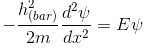# Problem: The Schrödinger equation for a free particle (no potential energy) is :What is the most general solution of the time-independent Schrödinger equation?a) Asin(kx)b) Asin(kx)+Bcos(kx)c) Ae-kx + Bekx

###### FREE Expert Solution

General solution refers to a wave equation before boundary conditions are applied.

87% (206 ratings)###### Problem Details

The Schrödinger equation for a free particle (no potential energy) is :What is the most general solution of the time-independent Schrödinger equation?

a) Asin(kx)
b) Asin(kx)+Bcos(kx)
c) Ae-kx + Bekx